# Ohmmeter: How Does it Work? (Series, Multi-Range & Shunt Type Ohm Meters)

Contents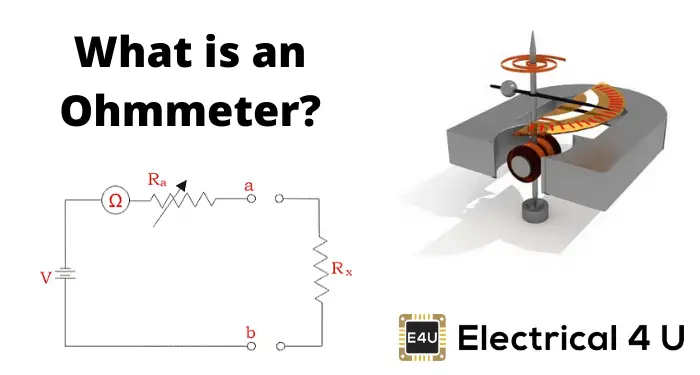## What is an Ohmmeter?

An ohmmeter (also known as an ohm meter) is an instrument that measures the electrical resistance of a material (resistance is a measure of the opposition to the flow of electric current). Micro-ohmmeters (micro ohmmeter or microhmmeter) and Milliohmmeters make low resistance measurements, while Megohmmeters (a trademarked device by Megger) measure large values of resistance.

Every device has electrical resistance. It may be large or small, and it increases with temperature for conductors and decreases with temperature for semiconductors.

There are many types of ohmmeters. Three of the most common ohm meters are:

1. Series ohmmeter.
2. Shunt ohmmeter.
3. Multi-range ohmmeter.

## Working Principle of Ohmmeter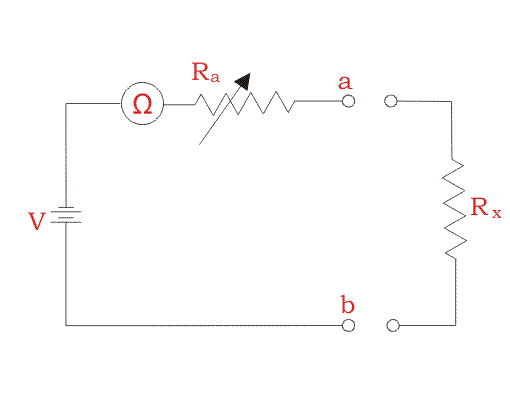The instrument is connected with a battery, a series adjustable resistor, and an instrument that gives the reading. The resistance to be measured is connected at terminal ob. When the circuit is completed by connecting output resistance, the circuit current flows and so the deflection is measured.

When the resistance to be measured is very high then the current in the circuit will be very small and the reading of that instrument is assumed to be the maximum resistance to be measured.
When resistance to be measured is zero then the instrument reading is set to zero position which gives zero resistance.

## D’Arsonval Movement

This type of movement is used in DC measuring instruments. The main principle in these types of instruments is that when a current-carrying coil is placed in a magnetic field, it feels a force and that force can deflect the pointer of a meter and we get the reading in the instrument.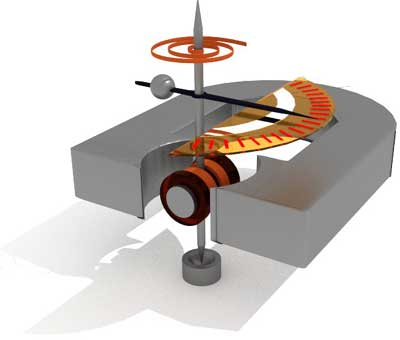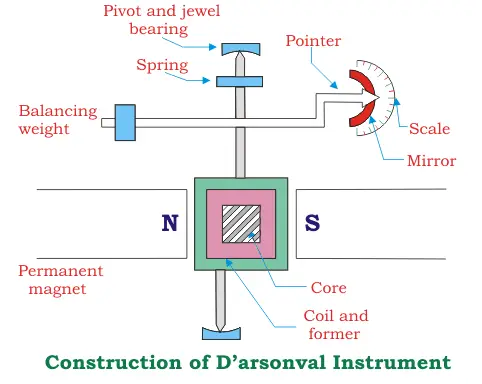This type of instrument consists of a permanent magnet and a coil that carries current and is placed in between them. The coil may be of rectangular or circular in shape. The iron core is used to provide a flux of low reluctance so it produces a high-intensity magnetic field.

Due to high-intensity magnetic fields, the deflecting torque produced is of large value due to which sensitivity of the meter is also increased. The current which entered comes out of two control springs, one in the upper side and one in the lower side.

If the direction of current is reversed in these types of instruments, then torque direction will also be reversed so these types of instruments are applicable in DC measurements only. The deflecting torque is directly proportional to the deflection angle hence these types of instruments have a linear scale.

To limit the deflection of the pointer we have to use damping which provides an equal and opposite force to the deflecting torque and hence the pointer comes to rest at a certain value.
The indication of the breeding is given by a mirror in which a beam of light is reflected on to the scale and hence deflection can be measured.

There are many advantages due to which we use the D’Arsonval type instrument. They are-

1. They have a uniform scale.
2. Effective eddy current damping.
3. Low power consumption.
4. No hysteresis loss.
5. They are not affected by stray fields.

Owing to posses those major advantages we can use this type of instrument. However, they suffer from drawbacks such as:

1. It cannot be used in alternating current systems (DC current only)
2. Costlier compared to MI instruments.
3. There may be an error due to the aging of springs by which we may not get accurate results.

However in the case of resistance measurement, we go for DC measurement because of the advantages offered by PMMC instruments and we multiply that resistance by 1.6 to find out AC resistance, so these instruments are much widely used due to their advantages. The disadvantages offered by it are dominated by the advantages so they are used.

## Series type Ohmmeter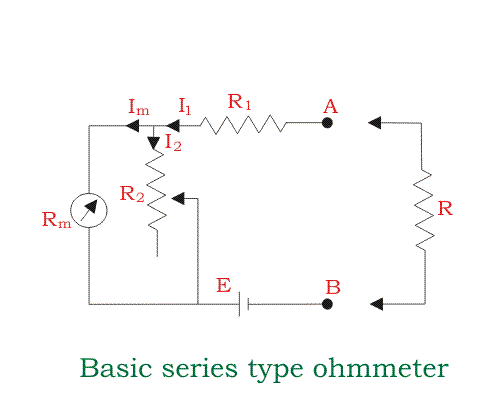The series type ohmmeter consists of a current limiting resistor R1, Zero adjusting resistor R2, EMF source E, Internal resistance of D’Arsonval movement Rm and the resistance to be measured R.
When there is no resistance to be measured, the current drawn by the circuit will be maximum and the meter will show a deflection.

By adjusting R2 the meter is adjusted to a full-scale current value since the resistance will be zero at that time. The co-responding pointer indication is marked as zero. Again when the terminal AB is opened it provides very high resistance and hence almost zero current will flow through the circuit. In that case, the pointer deflection is zero which is marked at a very high value for resistance measurement.

So a resistance between zeros to a very high value is marked and hence can be measured. So, when resistance is to be measured, the current value will be somewhat less than the maximum and the deflection is recorded and accordingly, resistance is measured.

This method is good but it posses certain limitations such as the decrease in the potential of the battery with its use so adjustment must be made for every use. The meter may not read zero when terminals are shorted, these types of problems may arise which is counteracted by the adjustable resistance connected in series with the battery.

## Shunt Type Ohmmeter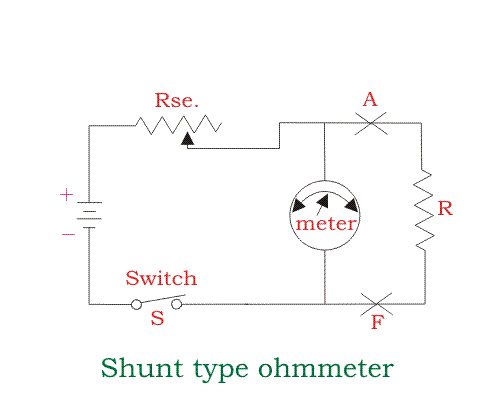In this type of meters, we have a battery source and an adjustable resistor is connected in series with the source. We have connected the meter in parallel to the resistance which is to be measured. There is a switch by the use of which we can on or off the circuit.

The switch is opened when it is not in use. When the resistance to be measured is zero, the terminals A and F are shorted so the current through the meter will be zero. The zero position of the meter denotes the resistance to be zero.

When the resistance connected is very high, then a small current will flow the terminal AF and hence full-scale current is allowed to flow through the meter by adjusting the series resistance connected with the battery.

So, full-scale deflection measures very high resistance. When the resistance to be measured is connected between A and F, The pointer shows a deflection by which we can measure the resistance values.

In this case, the battery problem may arise which can be counteracted by adjusting the resistance. The meter may have some error due to its repeated use also.

## Multi-Range Ohmmeter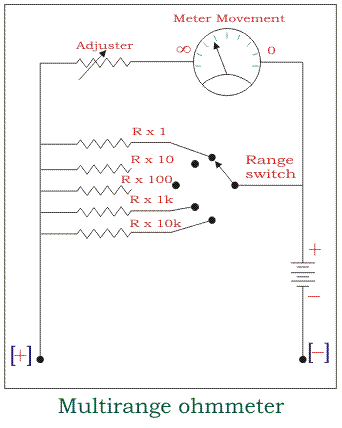This instrument provides the reading up to a very wide range. In this case, we have to select the range switch according to our requirements. An adjuster is provided so that we can adjust the initial reading to be zero.

The resistance to be measured is connected in parallel to the meter. The meter is adjusted so that it shows full-scale deflection when the terminals in which the resistance connected is full-scale range through the range switch.

When the resistance is zero or short circuit, there is no current flow through the meter and hence no deflection. Suppose we have to measure a resistance under 1 ohm, then the range switch is selected at the 1-ohm range at first.

Then that resistance is connected in parallel and the corresponding meter deflection is noted. For 1 ohm resistance, it shows full-scale deflection but for the resistance other than 1 ohm it shows a deflection which is less than the full load value, and hence resistance can be measured.

This is the most suitable method of all the ohmmeters as we can get an accurate reading in this type of meter. So this meter is most widely used nowadays.

Want To Learn Faster? 🎓
Get electrical articles delivered to your inbox every week.
No credit card required—it’s 100% free.